﻿ Calculated Boost Tutorial

#1. Introduction

The BoostSolutions Calculated Boost field allows users to do complex calculation based on other columns easily.

2. Create Calculated Boost column

a. Open the list or library where you want to apply the Calculated Boost feature. In the ribbon, click the List or Library tab. And click Create Column under the Manage Views group.

b. In the Name and Type section, type a column name of your choosing (e.g. Calculated Boost) and select Calculated Boost.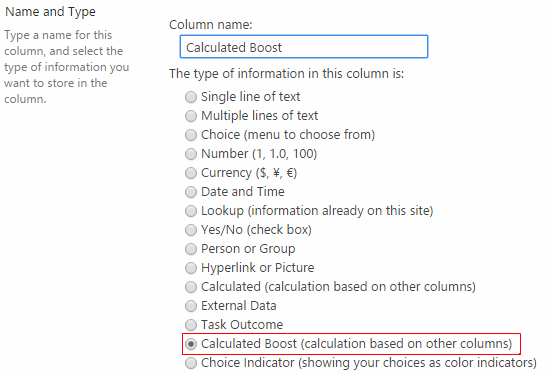c. In the formula box, build your calculation by columns, constants, functions and operators.

Select columns in Column list: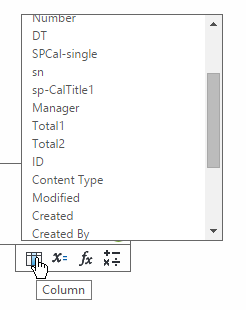Select Constants in Constants list: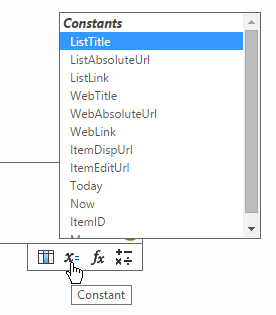Select functions in Function list: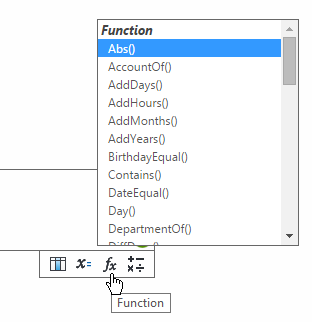Select operators in Operator list: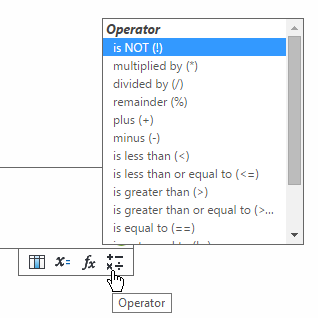For example, to calculate the average amount of column Total1 and Total2, you can build following formula, it is straight forward and easy-understanding:d. Select data type returned from this formulae. Select the checkbox to enable real-time calculation if necessary.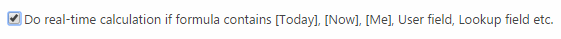3. Display the calculation result list